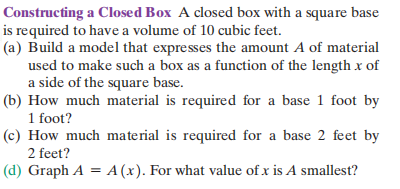### ¿Todavía tienes preguntas de matemáticas?

Pregunte a nuestros tutores expertos
Algebra
PreguntaA closed box with a square base is required to have a volume of $$10$$ cubic feet.

(a) Build a model that expresses the amount $$A$$ of material used to make such a box as a function of the length $$x$$ of a side of the square base.

(b) How much material is required for a base $$1$$ foot by $$1$$ foot?

(c) How much material is required for a base $$2$$ feet by $$2$$ feet?

(d) Graph $$A = A ( x )$$ . For what value of $$x$$ is $$A$$ smallest?

$$\left. \begin{array} { l } { \text { (a) } A ( x ) = 2 x ^ { 2 } + \frac { 40 } { x } } \\ { \text { (b) } 42 ft ^ { 2 } \text { (c) } 28 ft ^ { 2 } } \\ { \text { (d) } } \\ { A \text { is smallest when } x \approx 2.15 ft . } \end{array} \right.$$